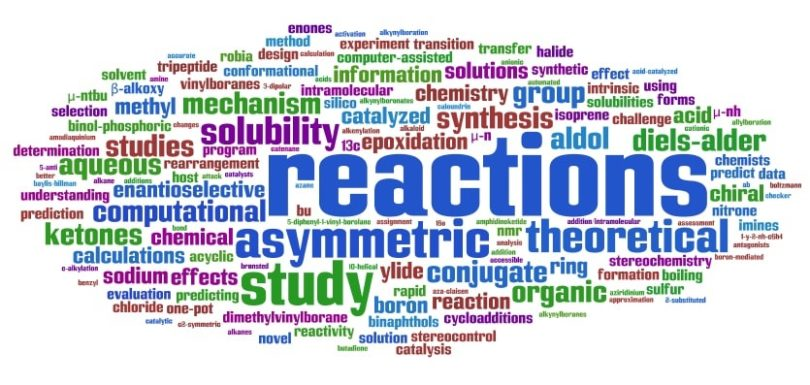Engineering Entrance Sample Papers

Alkali Metals Questions Answers for JEE MainsQues. The product obtained on fusion of BaSO4 and Na2CO3 is
(a) BaCO3
(b) BaO
(c) Ba(OH)2
(d) BaHSO4
Ans: (a)

Ques. On heating anhydrous Na2CO3, … is evolved
(a) CO2
(b) Water vapour
(c) CO
(d) No gas
Ans: (d)

Related: Chemistry KEAM Sample Papers

Ques. As compared to lithium, sodium reacts quickly with water because
(a) Its molecular weight is less
(b) It is stronger electronegative
(c) It is stronger electropositive
(d) It is  a metal
Ans: (c)

Ques. The most dangerous method of preparing hydrogen would be by the action of HCl and
(a) Al
(b) K
(c) Fe
(d) Zn
Ans: (b)

Ques. The reagent commonly used to determine hardness of water titrimetrically is
(a) Oxalic acid
(b) Disodium salt of EDTA
(c) Sodium citrate
(d) Sodium thiosulphate
Ans: (b)

Ques. Nelson cell is used for the preparation of
(a) Slaked lime
(b) Baryta
(c) Sodium
(d) Caustic soda
Ans: (d)

Ques. Which alkali metal is most metallic in character
(a) K
(b) Cs
(c) Na
(d) Li
Ans: (b)

Ques. The process of industrial manufacturing of sodium carbonate is known as
(a) Castner process
(b) Haber’s process
(c) Le-blanc process
(d) Chamber process
Ans: (c)

Ques. Alkali metals are
(a) Li, Na, Be, Mg, Cs
(b) Li, Na, K, Rb, Cs
(c) Na, K, Mg, Ca, Rb
(d) K, Rb, Cs, Ba, Sr
Ans: (b)

Ques. The alkali metal that reacts with nitrogen directly to form nitride is
(a) Li
(b) Na
(c) K
(d) Rb
Ans: (a)

Ques. In the Castner’s process for the extraction of sodium, the anode is made of…….metal.
(a) Copper
(b) Iron
(c) Sodium
(d) Nickel
Ans: (c)

Ques. Which has minimum solubility
(a) Br2S3
(b) Ag2S
(c) CoS
(d) PbS
Ans: (a)

Ques. Which one of the following is used as a disinfectant in water treatment?
(a) Alum
(b) Charcoal
(c) Kieselguhr
(d) Potassium permanganate
Ans: (d)

Ques. Which of the following properties is not true for an alkali metal
(a) Low atomic volume
(b) Low ionization energy
(c) Low density
(d) Low electronegativity
Ans: (a)

Ques. On dissolving moderate amount of sodium metal in liquid NH3 at low temperature, which one of the following does not occur
(a) Blue coloured solution is obtained
(b) Na+ ions are formed in the solution
(c) Liquid NH3 becomes good conductor of electricity
(d) Liquid ammonia remains diamagnetic
Ans: (d)

Ques. The word ‘alkali’ is used for alkali metals indicates
(a) Ash of the plants
(b) Metallic nature
(c) Silvery lusture
(d) Active metal
Ans: (a)

Ques. NaOH is prepared by the method
(a) Down’s cell
(b) Castner cell
(c) Solvay process
(d) Castner Kellner cell
Ans: (d)

Ques. Characteristic feature of alkali metals is
(a) Good conductor of heat and electricity
(b) High melting points
(c) Low oxidation potentials
(d) High ionization potentials
Ans: (a)

Ques. Photoelectric effect is maximum in
(a) Cs
(b) Na
(c) K
(d) Li
Ans: (a)

Ques. Electrolysis of molten sodium chloride leads to the formation of
(a) Na and H2
(b) Na and O2
(c) H2 and O2
(d) Na and Cl2
Ans: (d)

Ques. Which of the following metal has stable carbonates
(a) Na
(b) Mg
(c) Al
(d) Si
Ans: (a)

Ques. The colour given to the flame by sodium salts  is
(a) Light red
(b) Golden yellow
(c) Green
(d) Pink
Ans: (b)

Ques. The main salt soluble in sea water is
(a) MgCl2
(b) NaCl
(c) MgSO4
(d) CaSO4
Ans: (b)

Ques. When CO is passed over solid NaOH heated to 200oC, it forms
(a) Na2CO3
(b) NaHCO3
(c) HCOONa
(d) None
Ans: (c)

Ques. When NaOH is prepared, the gas released is
(a) Cl2
(b) H2
(c) O2
(d) H2O
Ans: (a), (b)

Ques. In the preparation of sodium carbonate, which of the following is used
(a) Slaked lime
(b) Quick lime
(c) Lime stone
(d) NaOH
Ans: (c)

Ques. Calcium is obtained by
(a) Roasting of limestone
(b) Electrolysis of solution of calcium chloride in H2O
(c) Reduction of calcium chloride with carbon
(d) Electrolysis of molten anhydrous calcium chloride
Ans: (d)

Ques. The colour of the precipitate produced by adding NaOH solution to HgCl2 is
(a) Yellow
(b) Black
(c) Brown
(d) White
Ans: (a)

Ques. The alum used for purifying water is
(a) Ferric alum
(b) Chrome alum
(c) Potash alum
(d) Ammonium alum
Ans: (c)